A dip needle lies initially in the magnetic meridian when it shows an angle of dip $\theta$ at a place. The dip circle is rotated through an angle x in the horizontal plane and then it shows an angle of dip $\theta \text{'}$ . Then  is

1. 1/cos x

2. 1/sin x

3. 1/tan x

4. cos x

Concept Questions :-

Earth's magnetism
High Yielding Test Series + Question Bank - NEET 2020

Difficulty Level:

A bar magnet has coercivity . It is desired to demagnetize it by inserting it inside a solenoid 12 cm long and having 60 turns. The current that should be sent through the solenoid is

1. 2 A                            2. 4 A

3. 6 A                            4. 8 A

Concept Questions :-

Curie law and hyterisis
High Yielding Test Series + Question Bank - NEET 2020

Difficulty Level:

For substances hysteresis (B - H) curves are given as shown in figure. For making temporary magnet which of the following is best.

(a)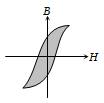(b)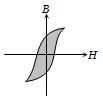(c)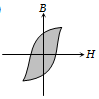(d)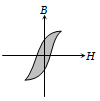Concept Questions :-

Curie law and hyterisis
High Yielding Test Series + Question Bank - NEET 2020

Difficulty Level:

The variation of magnetic susceptibility $\left(\chi \right)$ with temperature for a diamagnetic substance is best represented by

1.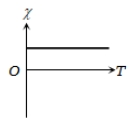2.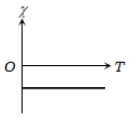3.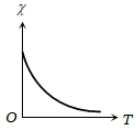4.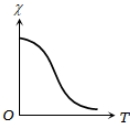Concept Questions :-

Magnetic materials
High Yielding Test Series + Question Bank - NEET 2020

Difficulty Level:

The variation of magnetic susceptibility $\left(\chi \right)$ with absolute temperature T for a ferromagnetic material is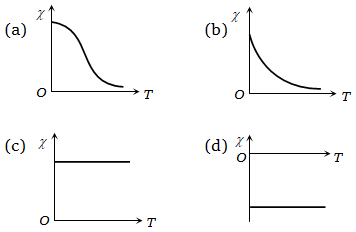Concept Questions :-

Curie law and hyterisis
High Yielding Test Series + Question Bank - NEET 2020

Difficulty Level:

The relative permeability $\left({\mu }_{r}\right)$ of a ferromagnetic substance varies with temperature (T) according to the curve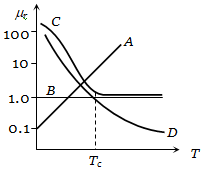(a) A                               (b) B

(c) C                               (d) D

Concept Questions :-

Magnetic materials
High Yielding Test Series + Question Bank - NEET 2020

Difficulty Level:

Which curve may best represent the current deflection in a tangent galvanometer ?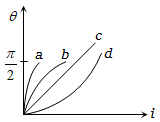(a) A                               (b) B

(c) C                               (d) D

Concept Questions :-

Magnetization and magnetic intensity
High Yielding Test Series + Question Bank - NEET 2020

Difficulty Level:

Some equipotential surfaces of the magnetic scalar potential are shown in the figure. The magnetic field at a point in the region is (X-axis read in cm)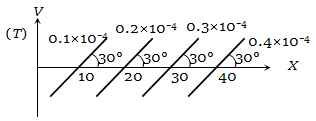(a)                         (b)

(c)                  (d) None of these

Concept Questions :-

Analogy between electrostatics and magnetostatics
High Yielding Test Series + Question Bank - NEET 2020

Difficulty Level:

The figure illustrates how B, the flux density, inside a sample of unmagnetized ferromagnetic material varies with B0, the magnetic flux density, in which the sample is kept. For the sample to be suitable for making a permanent magnet -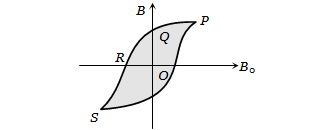1. OQ should be large and OR should be small

2. OQ and OR should both be large

3. OQ should be small and OR should be large

4. OQ and OR should both be small

Concept Questions :-

Curie law and hyterisis
High Yielding Test Series + Question Bank - NEET 2020

Difficulty Level:

The variation of the intensity of magnetisation (I) with respect to the magnetising field (H) in a diamagnetic substance is described by the graph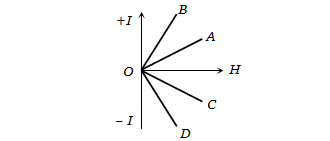1. OD                            2. OC

3. OB                            4. OA

Concept Questions :-

Magnetization and magnetic intensity